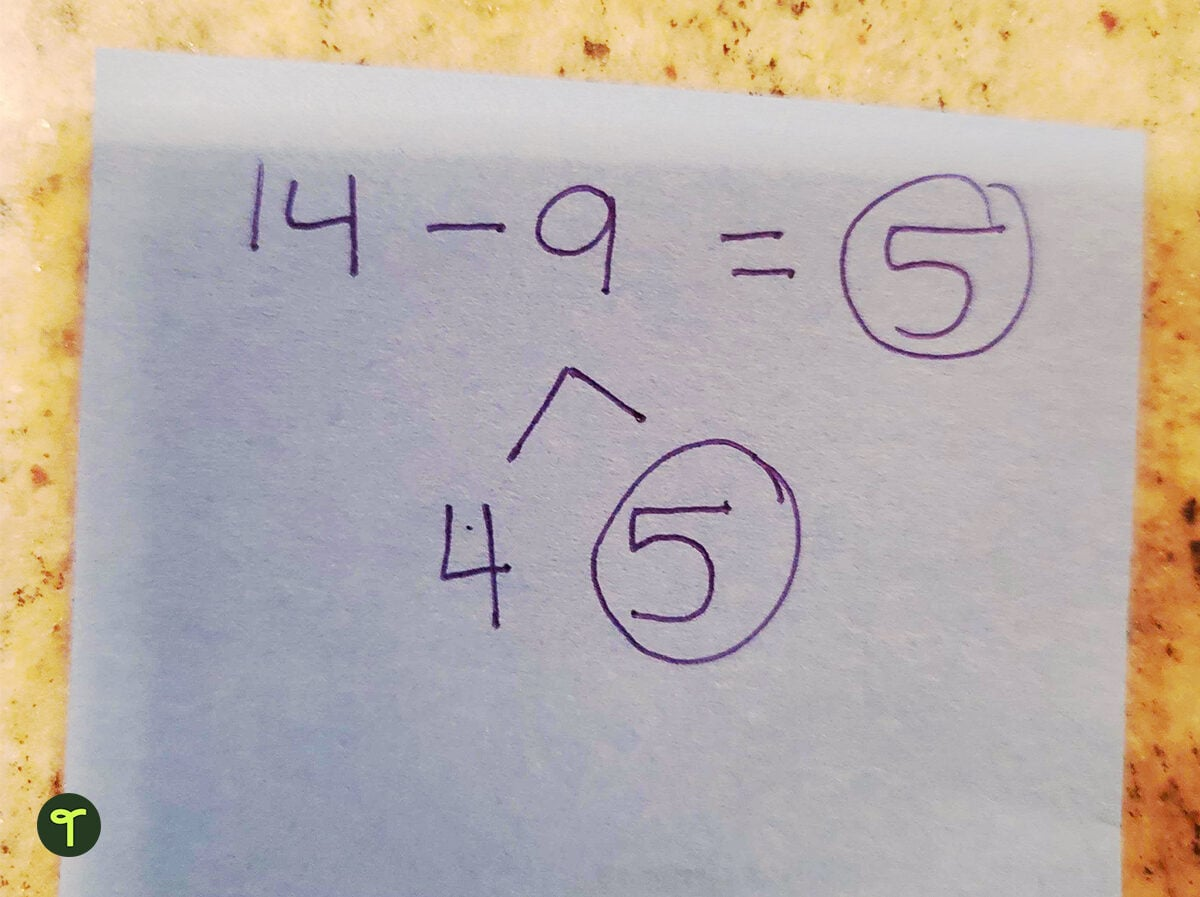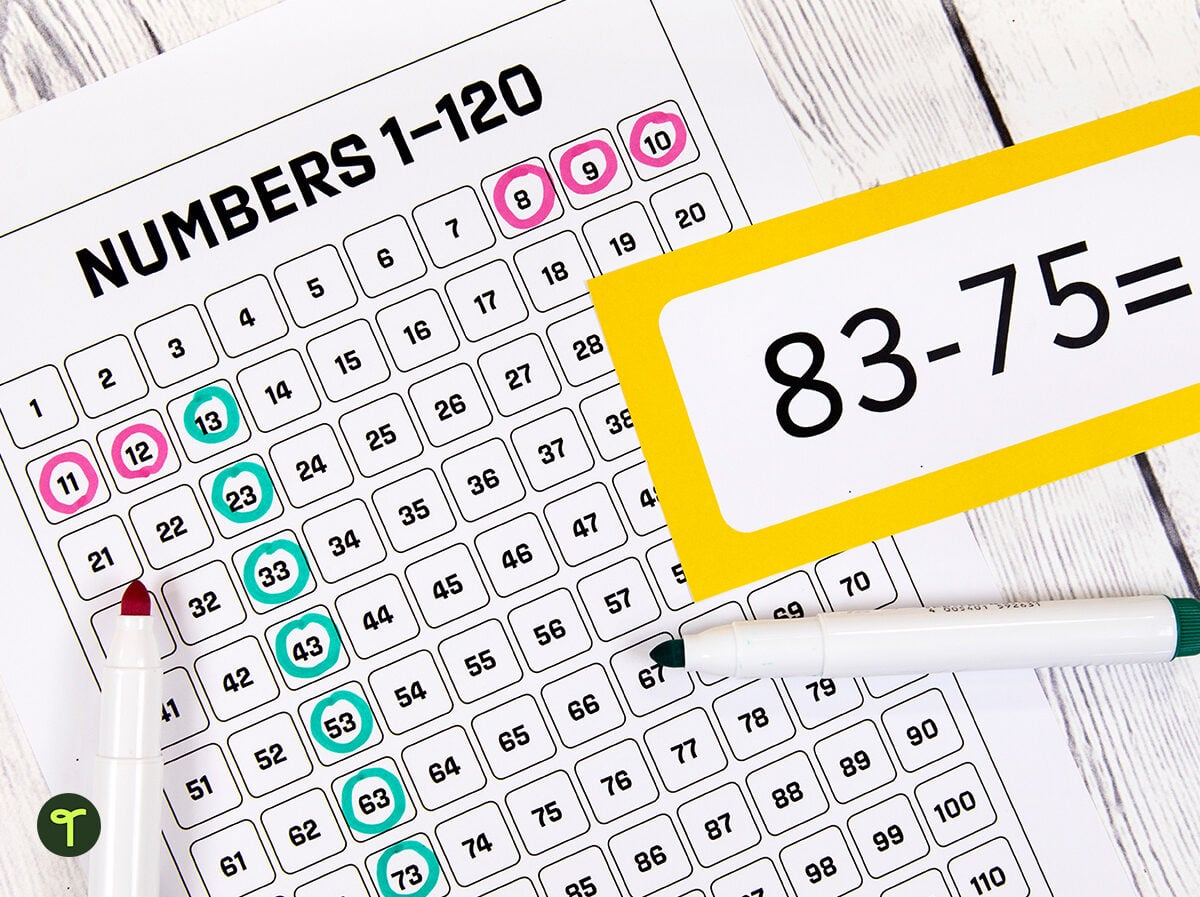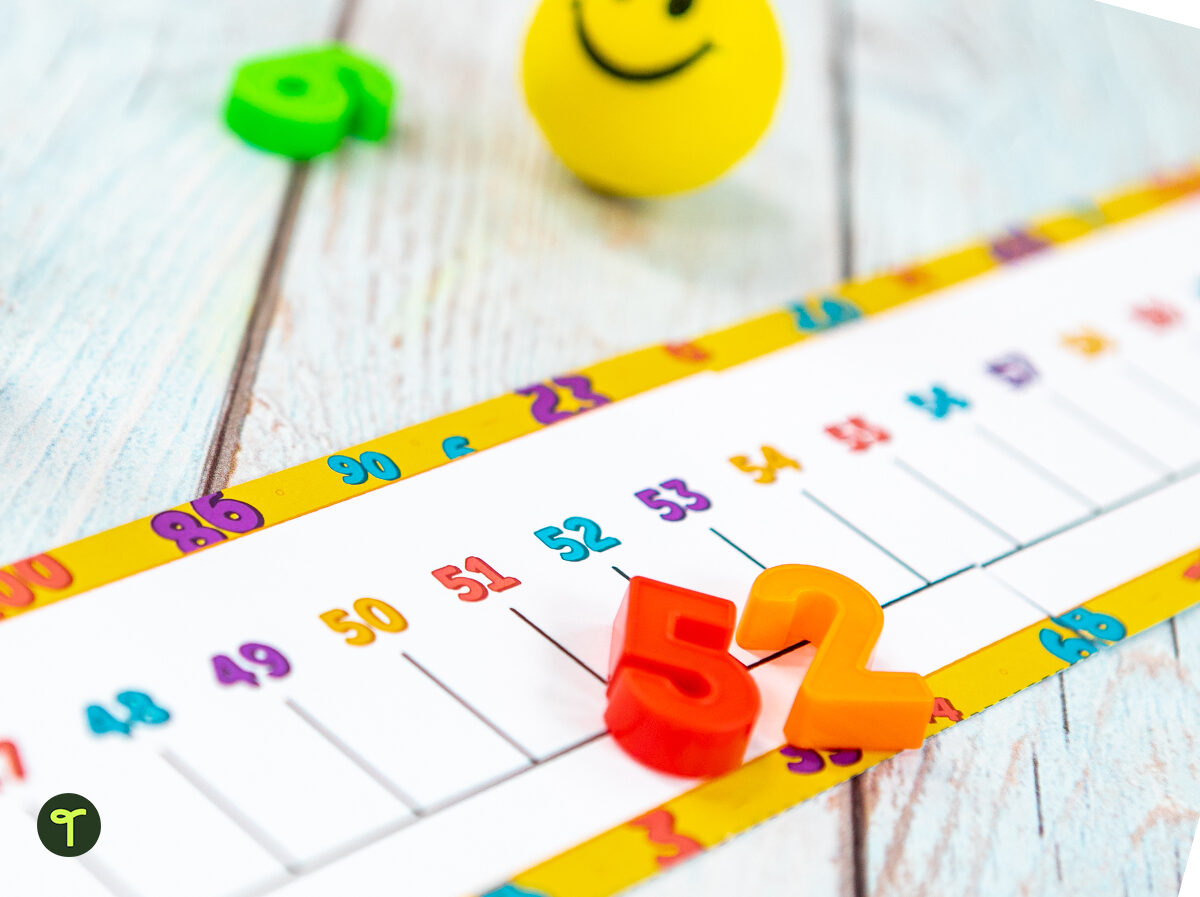# 6 Subtraction Strategies to Help Students Get Comfortable With Mental MathAh, subtraction strategies. Whether you’re new to teaching subtraction or looking for some new methods to add to your teacher toolbox, teaching young mathematicians different strategies to help them tackle a problem will not only boost their confidence in their mathematic skills now but also serve them as they grow and move on to more complicated math.

The math teachers on the teacher team at Teach Starter have taught a variety of subtraction strategies in our years in elementary classrooms, and we’ve put together this guide to help whether you’re brand-new to this part of the curriculum or just haven’t taught subtraction in a while. Feel free to explore and choose the strategies that work best for you and your learners.

## Why Do Kids Need Subtraction Strategies?

But wait a second, do students really need a bunch of strategies to subtract numbers? Is that really the best way to make sure kids meet the core standards? We assure you, there is a method to this madness!

Subtraction can be particularly challenging for young students, and that can be frustrating for teachers too. But this is not a knock on your abilities as a teacher. Research has long shown that young kids find subtraction harder than addition and some experts have called for less emphasis on subtraction fluency in the primary grades in favor of making sure students have a solid understanding of addition first.

Need subtraction worksheets? Get started with teacher-created options here

Researchers have also proven that students have substantially more success with subtraction when they can use their addition skills. Likewise, learners who understand the inverse relationship between addition and subtraction demonstrate better conceptual knowledge and — you guessed it — fare better with subtraction.

That’s where subtraction strategies play an important role in teaching this particular arithmetic operation. Rather than having to remember all their subtraction facts, students employ reasoning and their understanding of number relationships to come up with answers to the problems before them.

We know everyone learns differently, and students need to learn a variety of strategies as they develop their math fluency, so we’ve pulled together a host of options to help your students be more successful at subtraction.

## Subtraction Strategies

### Make Ten

Remember what we said about kids who understand the inverse relationship between addition and subtraction? The Make Ten subtraction strategy makes use of that relationship by reminding students that any subtraction problem can be reformed as a missing addend problem.

Many teachers teach students to break apart the larger number in a subtraction problem to “make 10.”

For example, say a student needs to solve 14-9. You might ask the student, “How can you change 14 into a ten? Take away 4.  This becomes the first number in your number bond for the number 9.  How many more do we need to make 9? (4 + ___ = 9) Five. Five is your answer.”Tip: Using ten frames and counters will help students to understand this strategy at first before they can move on to breaking down the math mentally. Download a fun ten frames match-up game!

If the numbers are near one another, students can simply “count up” from the number being subtracted (subtrahend) to the whole (minuend). This works best with numbers that are within 10 digits such as 456 and 459 or 21 and 27. Using word problems can help cement this particular subtraction skill.

### Use Doubles Facts

Another subtraction strategy that makes use of addition, using doubles plays off student memorization of doubles facts. For example, when a student remembers that 8 + 8 = 16, this can be used in reverse to deduce that 16 – 8 = 8.

### Use a Hundreds Chart

Teaching students to subtract two-digit numbers? Keep hundreds charts handy in your classroom for this subtraction strategy!

Let’s say you need to subtract 57-29. Find 53 on the chart. You know that 29 has 2 tens and 9 ones.

Start at 57 on the chart, then go back 2 tens, moving you to 37. Then count back 9 ones, moving you to 28.Snag an animated PowerPoint to use with students who are learning how to use the subtraction algorithm and borrowing.

### Split Strategy

Another popular mental math strategy for solving subtraction problems is known as the split strategy because — you guessed it — it involved splitting one of the numbers in the problem! In this case, students will split the second, smaller number (the subtrahend) into expanded form. This strategy will work with various subtraction problems — even those that need regrouping (more on that later).

So how does it work? Let’s say you have the subtraction problem 164-48.

The student will break the 48 down into its place value values or expanded form — 40 and 8.

Next, they’ll subtract 40 from 164, which can be done by counting back in 10s from 164 to 124. Finally, they subtract 8 to find the answer, 116.

This subtraction strategy is also often called the “break apart” strategy as you’re breaking apart your subtrahend into the tens and ones.

### Using a Number Line

Some students are visual learners, and using a number line is a subtraction strategy that plays to that strength! Sometimes called the “jump strategy,” this method allows students to subtract smaller numbers very easily. Students find the minuend on their number line and move toward the left according to the number given as the subtrahend, counting as they “jump” the space between them.As students develop in their skills, they can progress from counting the difference out by 1s to counting by 5s or 10s.

Tip: Provide each student with a number line that they can keep close by, along with a dry-erase sleeve to make the number line easily re-usable. Students can use a whiteboard marker to “jump” along the line to solve a variety of subtraction problems.

#### Get more subtraction strategy tools to teach this building block of math in your classroom, curated by the Teach Starter team of expert teachers.

Banner image via Shutterstock/Monkey Business Images

## Recommended resources

### 1 Comment

•